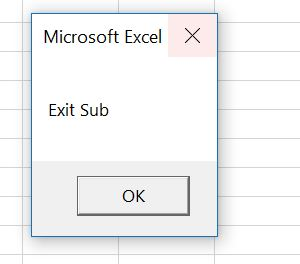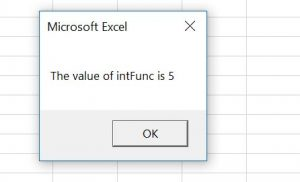# VBA Exit Sub or Function

In VBA, you can exit a Sub or Function, by using the Exit Sub or Exit Function commands.

``Exit Sub``
``Exit Function``

When the execution of the code comes to Exit Sub or Exit Function, it will exit a Sub or Function and continue with any other code execution.

If you want to learn how to exit a Sub on Error, click on this link: VBA On Error Exit Sub

If you want to learn how to end a whole code execution, click on this link: VBA End

## Exit a Sub in VBA

You will see on the example what happens when we use the Exit Sub command in a Sub. We created a Sub ExitSub, which has the Exit Sub command inside. The Sub CallExitSub calls this Sub. Here is the code:

``````Private Sub ExitSub()

Dim i As Integer

For i = 1 To 10
If i = 5 Then
Exit Sub
MsgBox "The value of i is" & i
End If
Next i

End Sub

Private Sub CallExitSub()
Call ExitSub
MsgBox "Exit Sub"
End Sub``````

In the ExitSub, we first enter the For Loop if the value of i is less than 10:

``````For i = 1 To 10

Next i``````

After that we check if the value of i is equal to 5, using the If command. If the value is 5, we want to exit the Sub and return the Message box with the value of i:

``````If i = 5 Then
Exit Sub
MsgBox "The value of i is" & i
End If``````

If the condition is not met, the following statement increases i by 1 and enters in the For loop again:

``Next i``

In the CallExitSub, we first call the Sub ExitSub:

``Call ExitSub``

After that we return the Message box:

``MsgBox "Exit Sub"``

If you run the CallExitSub, it will first call the ExitSub. If you execute this code in the debug mode, you will see that it will go through the loop 5 times. In the 5th iteration, the value of the variable i becomes 5 and the code enters in the If body. Now the Sub ExitSub is exited and returned to the CallExitSub. The next line is MsgBox “Exit Sub”:As you can see, the ExitSub is exited right after Exit Sub command, so the MsgBox “The value of i is” & i will be never executed.

## Exit a Function in VBA

Exiting a function in VBA is similar to exiting a Sub, just the command is Exit Function. In the example, we created the ExitFunc which returns an integer. The Sub CallExitFunction calls this function. Here is the code:

``````Private Function ExitFunc() As Integer

Dim i As Integer

For i = 1 To 10
If i = 5 Then
ExitFunc = i
Exit Function
End If
Next i

End Function

Private Sub CallExitFunction()
Dim intFunc As Integer
intFunc = ExitFunction()
MsgBox "The value of intFunc is " & intFunc

End Sub``````

In the ExitFunc, we first enter the For Loop if the value of i is less than 10:

``````For i = 1 To 10

Next i``````

After that we check if the value of i is equal to 5, using the If command. If the value is 5, we assign the value of i to the function result and exit the function:

``````If i = 5 Then
ExitFunc = i
Exit Function
End If``````

If the condition is not met, the following statement increases i by 1 and enters in the For loop again:

``Next i``

In the CallExitFunction, we first call the function ExitFunc: To do that we have to declare the variable intFunc type integer and assign the result of the ExitFunc function to it:

``````Dim intFunc As Integer

intFunc = ExitFunction()``````

After that we return the Message box with the value of intFunc:

``MsgBox "The value of intFunc is " & intFunc``

If you run the CallExitFunction, it will first call the function ExitFunc. If you execute this code in the debug mode, you will see that it will go through the loop 5 times. In the 5th iteration, the value of the variable i becomes 5 and the code enters in the If body. Now the value of the ExitFunc becomes i and the function is exited and returned to the CallExitFunction. The next line is MsgBox “The value of intFunc is ” & intFunc:Stop searching for VBA code online. Learn more about AutoMacro - A VBA Code Builder that allows beginners to code procedures from scratch with minimal coding knowledge and with many time-saving features for all users!